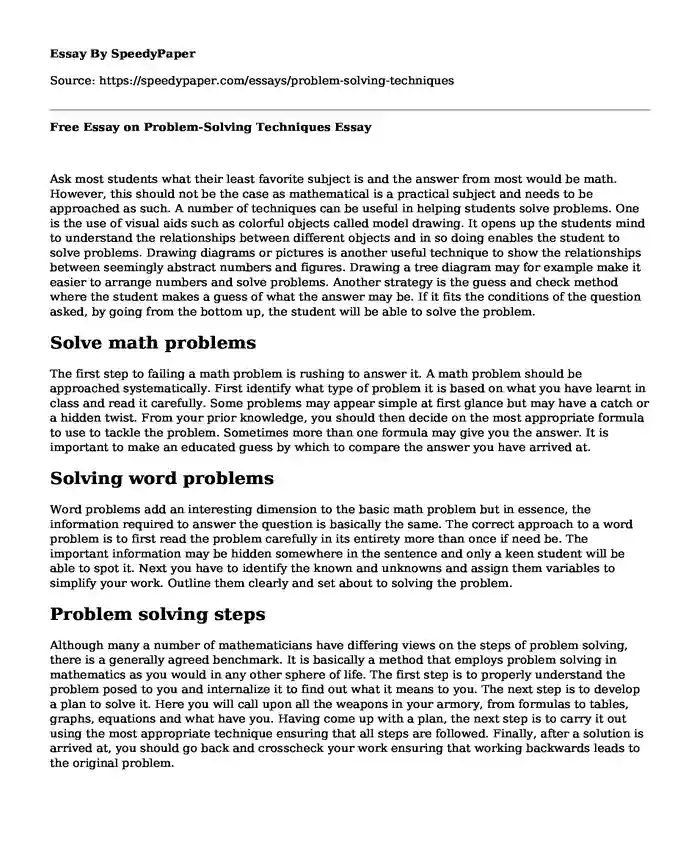# Free Essay on Problem-Solving Techniques

Published: 2019-11-18 14:47:48Categories: Problem solving Mathematics Pages: 2 Wordcount: 518 words
143 views

Ask most students what their least favorite subject is and the answer from most would be math. However, this should not be the case as mathematical is a practical subject and needs to be approached as such. A number of techniques can be useful in helping students solve problems. One is the use of visual aids such as colorful objects called model drawing. It opens up the students mind to understand the relationships between different objects and in so doing enables the student to solve problems. Drawing diagrams or pictures is another useful technique to show the relationships between seemingly abstract numbers and figures. Drawing a tree diagram may for example make it easier to arrange numbers and solve problems. Another strategy is the guess and check method where the student makes a guess of what the answer may be. If it fits the conditions of the question asked, by going from the bottom up, the student will be able to solve the problem.

Is your time best spent reading someone else’s essay? Get a 100% original essay FROM A CERTIFIED WRITER!

## Solve math problems

The first step to failing a math problem is rushing to answer it. A math problem should be approached systematically. First identify what type of problem it is based on what you have learnt in class and read it carefully. Some problems may appear simple at first glance but may have a catch or a hidden twist. From your prior knowledge, you should then decide on the most appropriate formula to use to tackle the problem. Sometimes more than one formula may give you the answer. It is important to make an educated guess by which to compare the answer you have arrived at.

## Solving word problems

Word problems add an interesting dimension to the basic math problem but in essence, the information required to answer the question is basically the same. The correct approach to a word problem is to first read the problem carefully in its entirety more than once if need be. The important information may be hidden somewhere in the sentence and only a keen student will be able to spot it. Next you have to identify the known and unknowns and assign them variables to simplify your work. Outline them clearly and set about to solving the problem.

## Problem solving steps

Although many a number of mathematicians have differing views on the steps of problem solving, there is a generally agreed benchmark. It is basically a method that employs problem solving in mathematics as you would in any other sphere of life. The first step is to properly understand the problem posed to you and internalize it to find out what it means to you. The next step is to develop a plan to solve it. Here you will call upon all the weapons in your armory, from formulas to tables, graphs, equations and what have you. Having come up with a plan, the next step is to carry it out using the most appropriate technique ensuring that all steps are followed. Finally, after a solution is arrived at, you should go back and crosscheck your work ensuring that working backwards leads to the original problem.